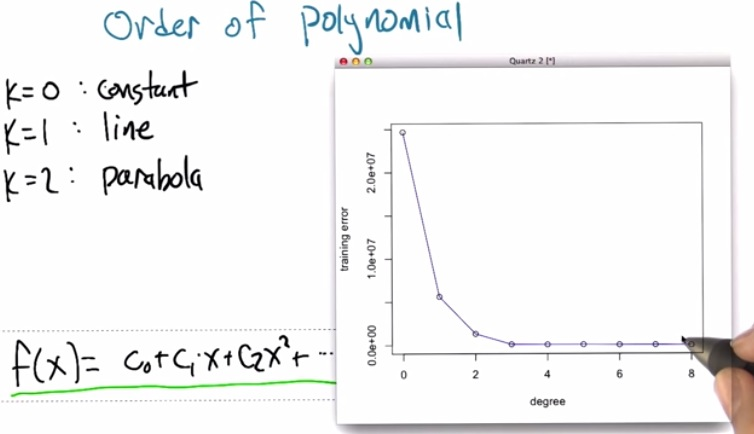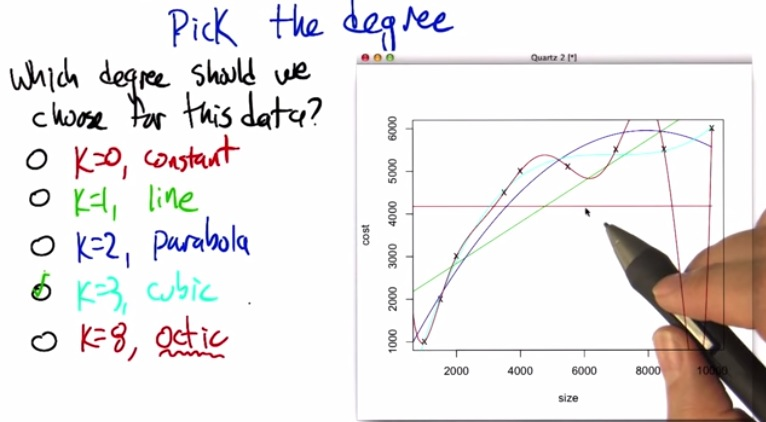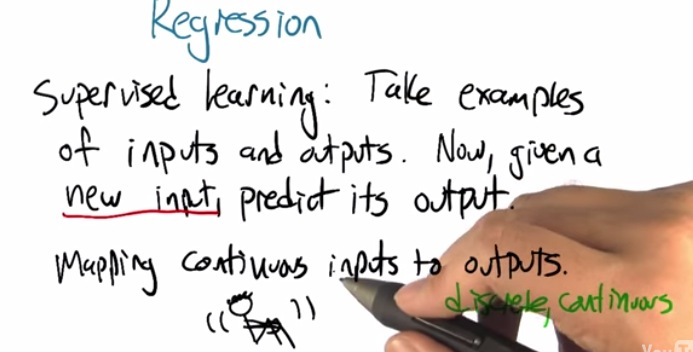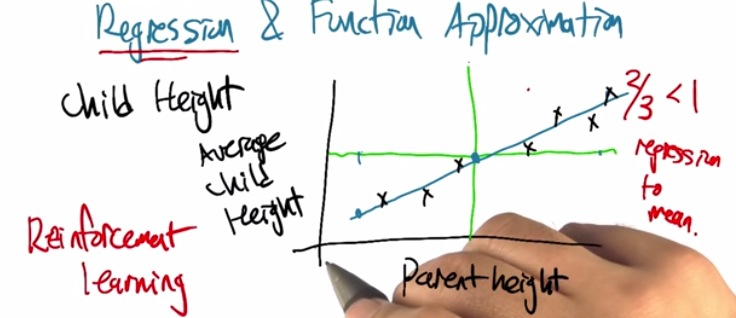• This the example where we want to predict the children's height based on the parent's height
• the horizontal line are avg child, and vertical is parent height.
• Based on existing data points, it drawn some linear line that approximate all data points.
• But earlier, terms of regression and reinforcement were used incorrrectly and has been stuck until now.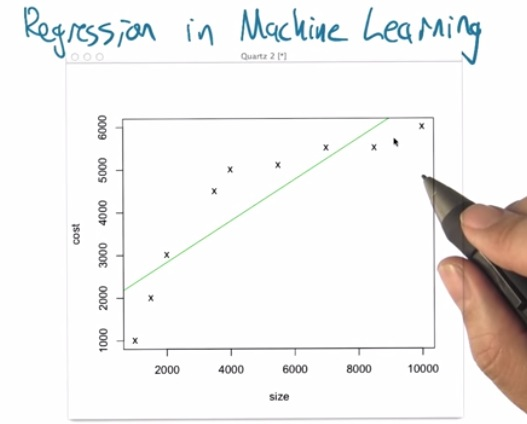• Linear regression maybe not the best case for predicting house sizes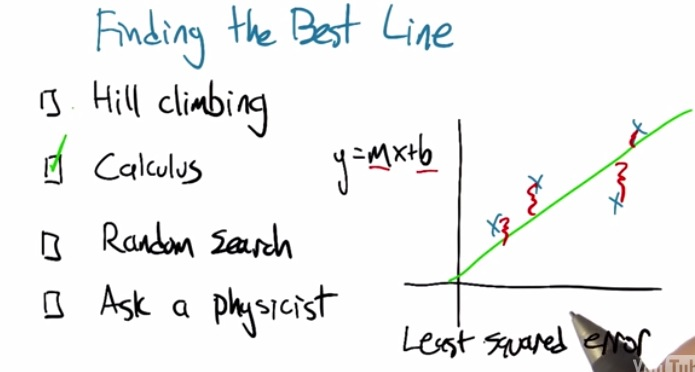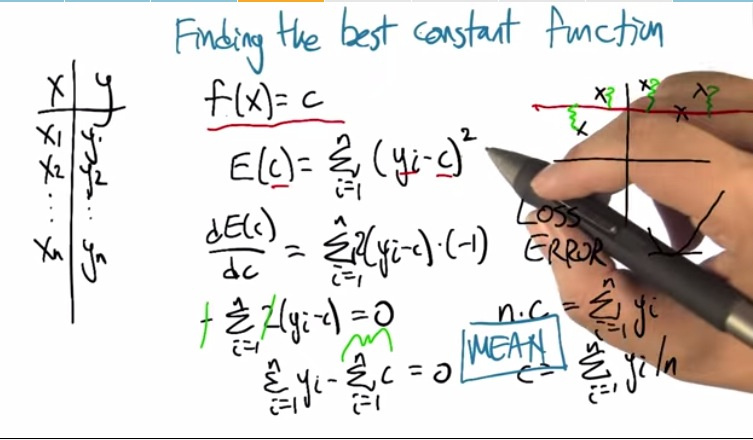• We want to the function approximation to always be constant, as shown in the red line on the right
• And we also want to find the minimum error,that based on the all error to c, what's the miniumum
• Then the we proceed it to the partial derrivative, with respect to c. As in calculus to get the minimum error, we set it equals to zero. That is, from the curve, if it reach lowest curve(~zero), we want to get what the point is
• (- and 2 are scrapped, is it because all the constant are nullify?)
• Then we sum c with n times, as c is always the same, then n.c, and thus produce the final result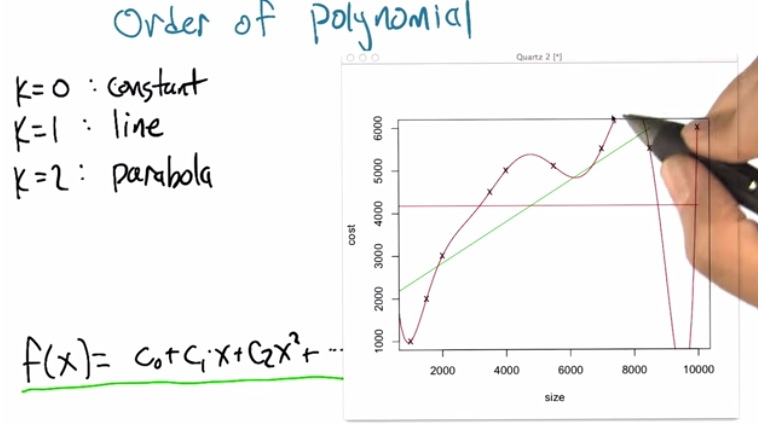• Here we can play the order of polynomial with little by little fit better everytime
• The order polynomial at 8, hit every single data points, and get the lowest error rate, as shown in also in the graph below
• Possible of overfitting?